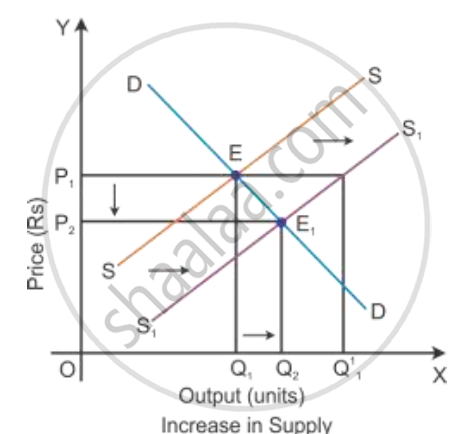Share

# Explain the Chain of Effects of Excess Supply of a Good on Its Equilibrium Price - CBSE (Arts) Class 12 - Economics

#### Question

Explain the chain of effects of excess supply of a good on its equilibrium price

#### Solution

Chain effects of excess supply of a good on its equilibrium price

Consider DD to be the initial demand curve and SS to be the supply curve of the market. Market equilibrium is achieved at Point E, where the demand and supply curves intersect each other. Therefore, the equilibrium price is OP, and the equilibrium quantity demanded is OQ.

When there is the change in other factors than price, there will be the rise in the supply of goods. There will be a shift in the supply curve towards the right to SS1 with an increase in the supply, and the demand curve DD will remain the same. This implies that there will be a
a situation of excess supply at the equilibrium pointIn the above diagram, there is an excess supply of OQ1 to OQ11 units of output at the initial price OP1. Thereby the producers will tend to reduce the price of the output to increase the sale in the market. A profit margin of the firm will come down and slowly some of the firms will tend to quit the market. Because of this, the market supply will decline to OQ2 level of output and the price of the output also gets reduced to the point OP2. Now, the new market equilibrium will be at Point E1, where the new supply curve SS1 intersects the demand curve DD.

Is there an error in this question or solution?

#### Video TutorialsVIEW ALL 

Solution Explain the Chain of Effects of Excess Supply of a Good on Its Equilibrium Price Concept: Equilibrium Price.
S